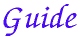# Definition 1

Those magnitudes are said to be commensurable which are measured by the same measure, and those incommensurable which cannot have any common measure.

###Two magnitudes A and B of the same kind are commensurable if there is another magnitude C of the same kind such that both are multiples of C, that is, there are numbers m and n such that nC = A and mC = B. See definition V.Def.5 for the definition of equality of ratios (also known as a proportion). If the two magnitudes are not commensurable, then they’re called incommensurable.

Propositions X.2 through X.8 and several later ones deal with commensurable and incommensurable magnitudes. In particular X.5 and X.6 state that two magnitudes are commensurable if and only if their ratio is the ratio of a number to a number. For example, if nC = A and mC = B, then the ratio of magnitudes A : B is the same as the ratio of numbers n : m. And conversely, if A : B = n : m, then the 1/nth part of A equals the 1/mth part of B.

Ratios of numbers are known to modern mathematicians as rational numbers while other ratios are known as irrational numbers. Unfortunately, Euclid used the words rational and irrational in a different way in Definition 3, see below.

# Definition 2

Straight lines are commensurable in square when the squares on them are measured by the same area, and incommensurable in square when the squares on them cannot possibly have any area as a common measure.

###Note that this definition only applies to lines, that is, only lines are ever said to be commensurable in square. Certainly, commensurable lines are also commensurable in square, but lines can be commensurable in square but not commensurable, in other words, commensurable is square only. The most famous example of this phenomenon consists of the side A and the diagonal B of a square. They are commensurable in square since the square on B is twice the square on A, by I.47. But they are not commensurable lines. In modern terms we would say that the square root of 2 is not a rational number.

# Definition 3

With these hypotheses, it is proved that there exist straight lines infinite in multitude which are commensurable and incommensurable respectively, some in length only, and others in square also, with an assigned straight line. Let then the assigned straight line be called rational, and those straight lines which are commensurable with it, whether in length and in square, or in square only, rational, but those that are incommensurable with it irrational.

###The proof referred to at the beginning of this definition is that of X.10 which finds lines commensurable in square only, and lines incommensurable in square.

Euclid uses the words rational and irrational differently than mathematicians both before and after him. The usual uses of these words correspond to commensurable and incommensurable, respectively. But when applied to lines, Euclid makes them correspond to commensurable in square and incommensurable in square. First, one line is chosen as a standard, then another line is called rational if it is commensurable in square, and irrational if not. Thus, the diagonal on the square on the standard line is rational, even though it’s incommensurable with the standard line, since it’s commensurable in square with it.

This confusing terminology makes Book X difficult to understand.

# Definition 4

And the let the square on the assigned straight line be called rational, and those areas which are commensurable with it rational, but those which are incommensurable with it irrational, and the straight lines which produce them irrational, that is, in case the areas are squares, the sides themselves, but in case they are any other rectilineal figures, the straight lines on which are described squares equal to them.

###Although Euclid uses rational in an unusual way for lines, he uses it in the usual way for areas, so that an area is rational, according to Euclid, if it’s commensurable with the standard square, and irrational otherwise.